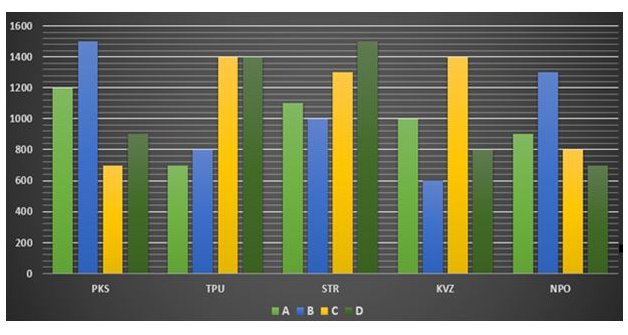# SBI Clerk Prelims 2021 Quantitative Aptitude Questions (Day-64)

Dear Aspirants, Our IBPS Guide team is providing new series of Quantitative Aptitude Questions for SBI Clerk Prelims 2020 so the aspirants can practice it on a daily basis. These questions are framed by our skilled experts after understanding your needs thoroughly. Aspirants can practice these new series questions daily to familiarize with the exact exam pattern and make your preparation effective.

Start Quiz

Bar graph

Directions (01-05): Study the following information carefully and answer the questions given below:

The following graph represents the number of four different products manufactured by five different companies in 2015.1) Total number of product A manufactured by all the companies in 2015 is what percent more/less than the total number of product D manufactured by all the companies in 2015.

A.6.85% less

B.7.54% less

C.6.85% more

D.7.54% more

E.none of these

2) Number of product B manufactured by STR in 2016 is 30% more than that of in 2015 and the number of product C manufactured by KVZ in 2016 is 20% less than that of in 2015. Find the respective ratio of number of product B manufactured by STR in 2016 and number of product C manufactured by KVZ in 2016.

A.56:65

B.45:54

C.65:56

D.54:45

E.None of these

3) Find the difference between total number of products manufactured by STR in 2015 and total number of products manufactured by NPO in 2015.

A.1200

B.1800

C.2400

D.2800

E.none of these

4) If the production of products A, B, C and D by TPU in 2016 is 20%, 30%, 40% and 50% respectively more than those of 2015, find the sum of production of all the products by TPU in 2016.

A.5490

B.4950

C.5940

D.4590

E.None of these

5) Number of product C manufactured by TPU in 2016 is 25% more than that of 2015 and number of product C manufactured by TPU in 2017 is 10% less than that of 2016. Number of product A manufactured by NPO in 2016 is 10% less than that of 2015 and number of product A manufactured by NPO in 2017 is 30% more than that of 2016. Number of product C manufactured by TPU in 2017 is what percent of number of product A manufactured by NPO in 2017?

A.108.67%

B.135.52%

C.112.45%

D.149.57%

E.None of these

Simplification

Directions (6-10): What will come in place of question mark (?) in the following question?

6) 45879 + 24856 + 1245 + 214 – 101 =?

A.72093

B.18581

C.26548

D.65412

E.96547

7) √10404 + √3844 + √529 =?

A.489

B.458

C.187

D.245

E.189

8) 2565 + 2700/12 – 895 + 302 = 3√?

A.2197

B.13

C.2456

D.14

E.None of these

9) 19/28 of 35% of 3416 =? x 610

A.1.33

B.2.12

C.1.89

D.2.18

E.0.58

10) 80 * 41 – 17 * 52 = (?)2 * 6 + 860

A.25

B.81

C.22

D.16

E.20

Total number of product A manufactured by all the companies in 2015

= 1200 + 700 + 1100 + 1000 + 900

= 4900

Total number of product D manufactured by all the companies in 2015

= 900 + 1400 + 1500 + 800 + 700

= 5300

Required percentage = (5300 – 4900)/5300 x 100

= (400/5300) x 100

= 7.54% less

Number of product B manufactured by STR in 2016 = 1000 x 130/100 = 1300

Number of product C manufactured by KVZ in 2016 = 1400 x 80/100 = 1120

Required ratio = 1300 : 1120 = 65:56

Total number of products manufactured by STR in 2015

= 1100 + 1000 + 1300 + 1500

= 4900

Total number of products manufactured by NPO in 2015

= 900 + 1300 + 800 + 700

= 3700

Required difference = 4900 – 3700 = 1200

Sum of production of all the products by TPU in 2016

= 700 x 120/100 + 800 x 130/100 + 1400 x 140/100 + 1400 x 150/100

= 840 + 1040 + 1960 + 2100

= 5940

Number of product C manufactured by TPU in 2017

= 1400 x 125/100 x 90/100

= 1575

Number of product A manufactured by NPO in 2017

= 900 x 90/100 x 130/100

= 1053

Required percentage = (1575/1053) x 100 = 149.57%

45879 + 24856 + 1245 + 214 – 101 =?

72194 – 101 =?

=72093

√10404 + √3844 + √529 =?

102 + 62 + 23 = 187

2565 + 2700/12 – 895 + 302 = 3√?

2565 + 225 – 895 +302 = 3√?

2197 = 3√?

? = 13

(19/28) * (35/100) *3416 =? * 610

(19/28) * (7 * 5/100) * (28 * 122) =? * 5 * 122

? = (19 * 7 * 5 * 122)/(5* 122 * 100)

? = (19 * 7)/100 = 1.33

(?)2 * 6 = 80 * 41 – 17 * 52 – 860

(?)2 * 6 = 3280 – 884 – 860 = 1536

(?)2 = 256

? = 16

 Check Here to View SBI Clerk Prelims 2021 Quantitative Aptitude Questions Day – 63 Day – 62 Day – 61